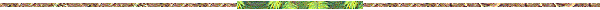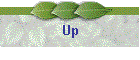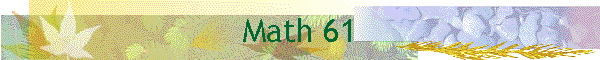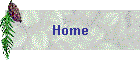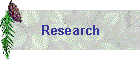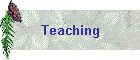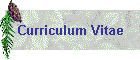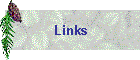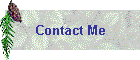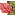Course: Math 61, Introduction to Discrete StructuresText: R. Johnsonbaugh, Discrete Mathematics, 5th Ed., Prentice-HallSchedule of Lectures: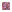Section 1.6: Mathematical inductionSection 2.4: RelationsSection 2.5-6: Equivalence relations, matrices of relationsSection 2.8: FunctionsSection 4.1: Basic counting principlesSection 4.2: Permutations and combinationsSection 4.6: Generalized permutations and combinationsSection 4.7: Binomial coefficientsSection 4.8: Pigeonhole principleSection 5.1: Recurrence relationsSection 5.2: Solving recurrence relations (including material in exercises 40-46)Section 6.1: Examples of graphsSection 6.2-3: Paths and cyclesSection 6.4: Shortest-path algorithmSection 6.5: Representation of graphsSection 6.6: Representation of graphsSection 6.7: Isomorphism of graphsSection 7.1: Examples of treesSection 7.2: More TreesSection 7.3-4: Minimal spanning treesSection 7.5: Binary treesSection 5.3, 7.7: Sorting (including merge sort from 5.3)Section 7.8: Isomorphic trees